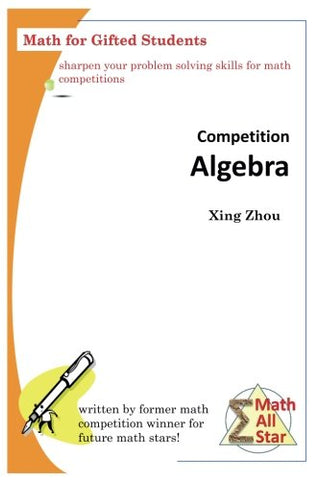# Competition Algebra: Math for Gifted Students (Math All Star)

• ISBN-13: 9781542567121
• \$31.04

Only 3 left!

Algebra is taught from elementary school to college and beyond. Algebraic problems present a significant portion in all math competitions including MathCounts, AMC, AIME, USAMO and so on. Therefore, solving competition level algebraic problems is a must-master skills for every contest contender. Algebra includes a wide range of topics and techniques. Some of them may be related to advanced mathematical theorems and tools. Therefore, it is impossible to cover all of them in one book. However, middle school and high school level competitions usually do not require advanced mathematics. Instead, the emphasis is on the applications of basic algebraic skills in a flexible and effective way to solve complex problems. As a result, it is a wise strategy to thoroughly understand the most important topics and drill down into details of related solving techniques in order to improve one's skill and test performance. This book covers three basic but important topics: equation, sequence and function. While these topics are all taught in schools, there are some competition specific techniques which deserve a systematic discussion. Taking Vieta's theorem as an example. While polynomial transformation is a well known method to evaluate expressions such as \$x_1^2+x_2^2\$, there are several other powerful techniques. They can be used to evaluate some complex expressions in a more efficient and less error-prone way. These expressions can have high power such as \$x_1^{7}+x_2^{7}\$, or are asymmetric such as \$5x_1^3 + 3 x_2^5\$. In fact, the latter asymmetric expression can present a challenge to many students who only know the polynomial transformation method. In addition to expression evaluation, Vieta's theorem can also be used to solve some seemingly unrelated problems. Such problems are among top hits in various math competitions. Sequence is another good example. Most students understand the two basic types of sequences, namely, arithmetic and geometric. Though the vast majority of sequence related problems in math contests can be converted to these basic types, finding such conversion may be a demanding task which is usually not discussed in classrooms. Meanwhile, in order to become a strong competitor, one must also understand a few additional more complex sequences especially those defined recursively. They are beyond the scope of school textbooks, but are discussed in this book. The goal of this book is to give an organized in-depth discussion on competition level techniques. Fully understanding these techniques will help students to quickly recognize and solve these types of problems. It will also lay down a solid foundation for them to solve other problems whose solutions require these algebraic techniques as critical stepping stones. Please visit http://www.mathallstar.org/ for more information.